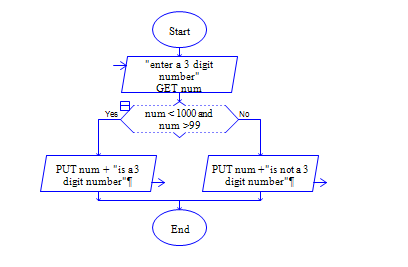# Java Program to find whether the given number is 3 digit number or not

Get a number num and check whether num is three digit number or not?.

Sample Input 1:

1000

Sample Output 1:

Not a three digit number

Sample Input 2:

987

Sample Output 2:

Three digit number

#### Flow Chart Design#### Program or Solution

``` import java.util.*; class ThreeD { public static void main(String args[]) { int num; Scanner sc=new Scanner(System.in); System.out.println("Enter The Number:"); num=sc.nextInt(); if(num>99 && num<1000) { System.out.println("It is a Three Digit Number."); } else { System.out.println("It is Not a Three Digit Number."); } } } ```

#### Program Explanation

1. Get input num from user using Scanner Class

2. check whether the num is greater than 99 and less than 1000 using if statement.

if it is true, then

2a. print num is three digit number using println function.

else

2b. print num is not three digit number using println function.